# What is Equivalent Radiated Power (ERP)?

## Definition

Given the normal vibration velocity of an elementary surface, it is possible to evaluate the acoustic power with the following relation: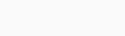where:

•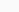is the overall acoustic power [W] radiated by the system under study (e.g. an electric powertrain)
•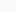is the air volumetric mass density [kg/m^3]
•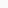is the sound velocity in air [m/s]
•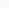is the normal vibration velocity [m/s2] of the surface
•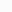is the surface area [m^2]
•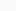is the radiation factor []

In the case of the Equivalent Radiated Power model, the elementary surface is assumed to radiate like a plate with a unit efficiency: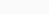## Application to e-NVH

In detailed design phase, the system-level geometry and structural parameters are known. A 3D FEA mechanical model can therefore be built and the structural dynamics of the e-machine can be represented by its modal basis. Based on the electromagnetic forces applied on the structure and the associated modal basis, the vibration of the outer enveloppe nodes of the system can be computed for each mode. ERP is then used to quickly evaluate the radiated acoustic power of the machine.

## Application to Manatee

In Manatee software, ERP model can be used to compute the radiated acoustic power of a machine when a modal basis from a 3D FEA mechanical model is used for structural computations.

When an analytical model is used for structural mechanics based on equivalent cylindrical shell equations, Manatee also uses an analytical estimation of the radiated power level which does not assume a simple unit radiation efficiency.

## Limitations

By using an unitary radiation factor, ERP is known to overestimate the acoustic radiation at low frequencies.
The following figure illustrates ERP model against acoustic FEA (coupling of Manatee with Actran). Resonances occuring at “low frequency” using ERP should be considered carefully.